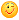About Store Forum Documentation ContactLerping at constant speeds Author Message
RubeusMemberPost: #1 Lerping at constant speeds I have an issue. I need to interpolate from point x to y to z at a constant speed. When just adding Time.d( ) to the step, it essentially finishes the traversal in 1 second; this is nice, but then if x to y is a length of .5 and y to z is a length of 100, there's a quite significant speed difference. But by dividing Time.d( ) by the length between the points being traversed, I get a uniform speed as I desired. Yaaaay. But now I'm trying to work with the Hermite Spline (Lerp4). The distance between x and y is not the same as the path it traverses due to the arcing and such. Dividing by the distance results in *very* noticeable jumps in speed when switching from x->y to y->z. I looked around online to see if there was some kind of solution I could understand(There's not.) I was wondering if any of you that are better than me with the black magic that is Calc/Trig knew of a solution that could help me out. Note that I *could* do a preliminary run through the Lerp4 using a set step (such as .1 or so) and add together the lengths of all the resulting vectors to get something of an approximation. But this seems computationally ridiculous to me, and would only use this method as a last resort.
07-13-2014 07:40 PM
SamNainocardMember

 Post: #2 RE: Lerping at constant speeds I'm not sure about Lerp4 (never used), but have you tried something like speed/sec or time needed to travel? something like these? it's what I used though. Code: // convert speed/sec to time needed to travel (so it will move at desired_speed) T1=t1=Dist(x, y)/desired_speed; T2=t2=Dist(y, z)/desired_speed; /* // reach the end at specified time T1=t1=5.0; T2=t2=5.0; */ t1-=Time.d(); t2-=Time.d(); Lerp (    y, x,     Sat(t1/T1)); Lerp (    z, y,     Sat(t2/T2)); Lerp4(.., y, x, .., Sat(t1/T1)); Lerp4(.., z, y, .., Sat(t2/T2));
07-13-2014 09:10 PM
RubeusMemberPost: #3 RE: Lerping at constant speeds That's very similar to what I was doing; the problem is that Lerp4 is a hermite spline- meaning there is a high probability of the returned coordinates not being between fromPoint and toPoint. In fact, it could be an 'S' shape. In this case, Dist(fromPoint, toPoint) is often too small a value to represent the actual path. (The hermite spline is essentially a way of calculating a Bezier curve, if you are more familiar with that term.)
07-13-2014 10:01 PM
EsenthelPost: #4 RE: Lerping at constant speeds I know what you mean, but I don't have any idea how to solve this07-14-2014 12:21 AM
EsenthelRubeus